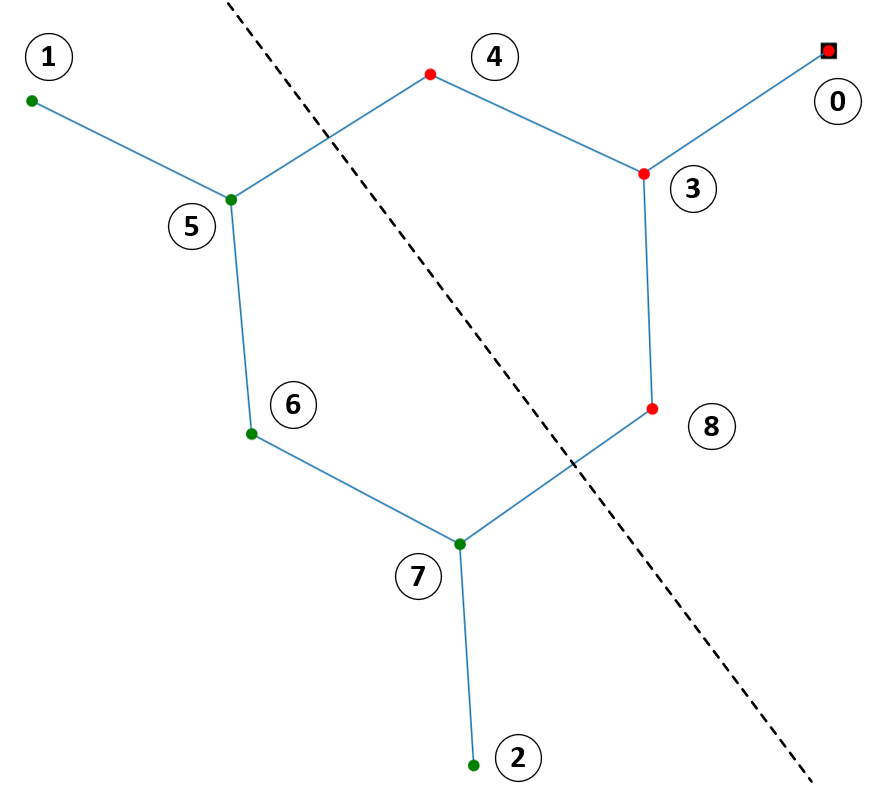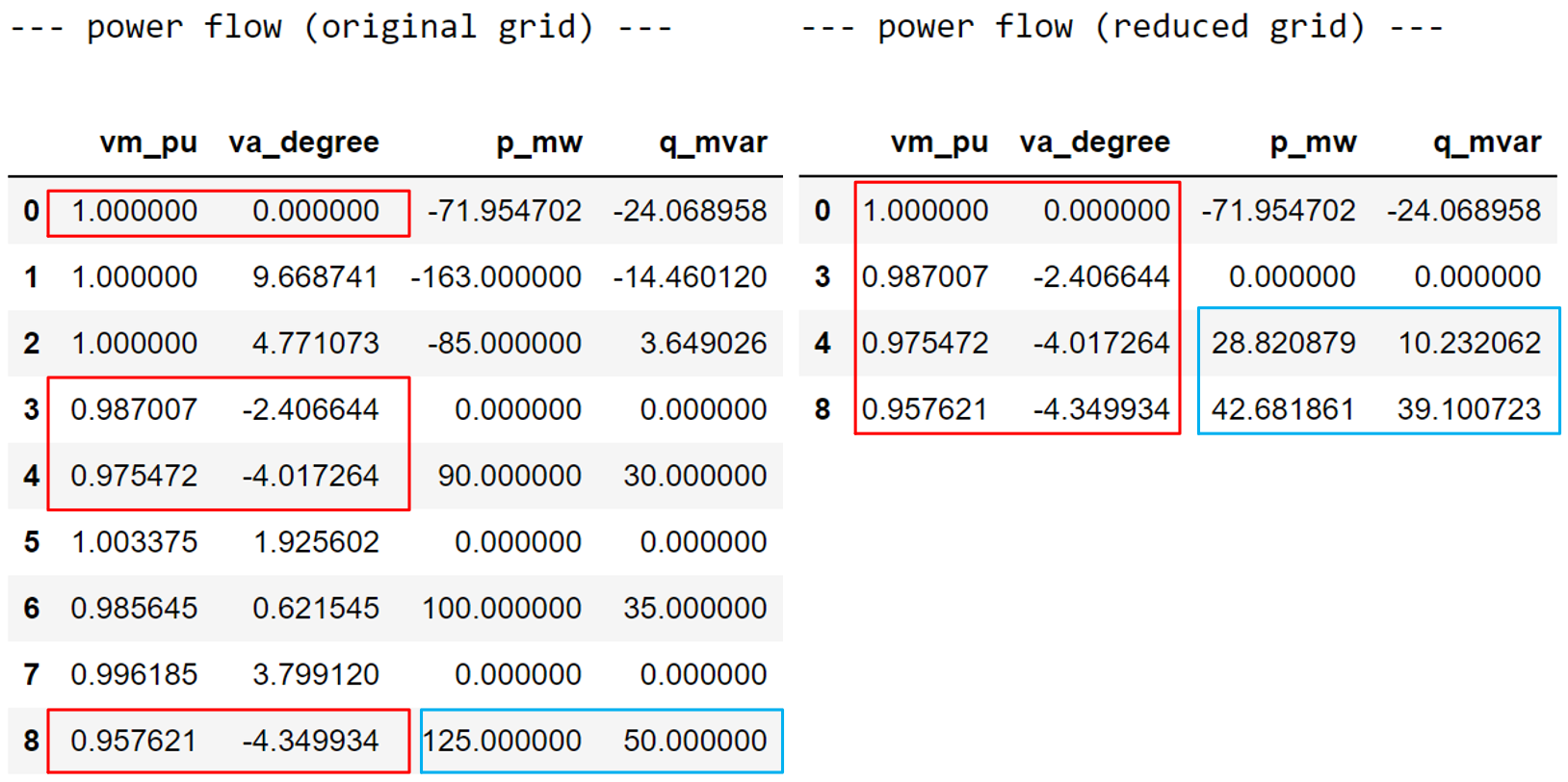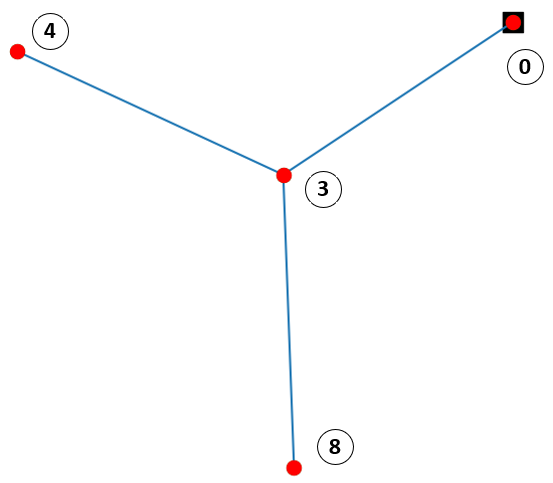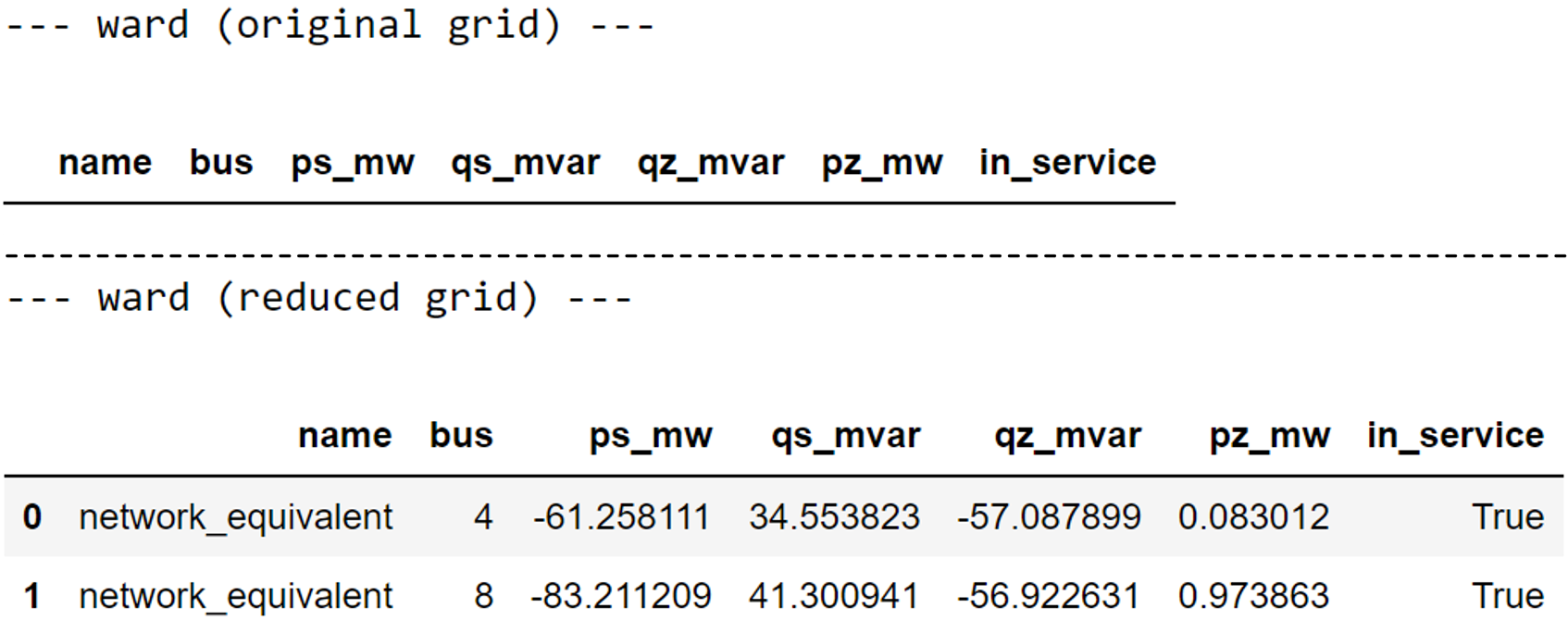# Grid Equivalent Example

Here is an example of what you can do:

## Create Example Network

First, we create an example network.

import pandapower as pp

net = pp.case9()

# getting power flow results
pp.runpp(net)


## Define Grid Areas

The created network is shown below. Assuming that the buses (red) [0, 3, 4, 8] belong to the internal subsystem which should be remained, and the external subsystem consists of buses (green) [1, 2, 5, 6, 7] that are going to be reduced. The boundary buses [4, 8] are belonging to the internal subsystem.## Execute Grid Equivalent Calculation

According to the above assumptions, input variables for the Ward-equivalent are:

# equivalent type
eq_type = "ward"

# internal buses: we don't need to give all internal buses to the function. Just one of them is enough.
internal_buses = 

# boundary buses
boundary_buses = [4, 8]


Then we start the Ward-equivalent calculation:

net_eq = pp.grid_equivalents.get_equivalent(net, eq_type, boundary_buses, internal_buses)


## Power Flow Results Comparison

We can compare the power flow results between the original grid “net” and the reduced grid “net_eq”:

print("--- power flow (original grid) ---")
net.res_bus
print("--- power flow (reduced grid) ---")
net_eq.res_busIt can be seen that the power flow results (vm_pu, va_degree) of the internal buses [0, 3, 4, 8] in both grids are the same (the difference is smaller than $$10^{-6}$$ pu or degree), i.e., the equivalent calculation is successful. The p_mw and q_mvar values at the boundary buses [4, 8] are different due to the created Ward elements. The figure below shows the reduced grid.## Equivalent Elements

We can print the calculated Ward elements using:

print("--- ward (original grid) ---")
net.ward
print("--- ward (reduced grid) ---")
net_eq.wardNote

If you compare the resulting (x)ward-parameters between pandapower and PowerFactory, you will see they are different. This is because in PowerFactory the admittance matrix is reconstructed according to a voltage sensitivity analysis, which is not open-source and leads to the difference.